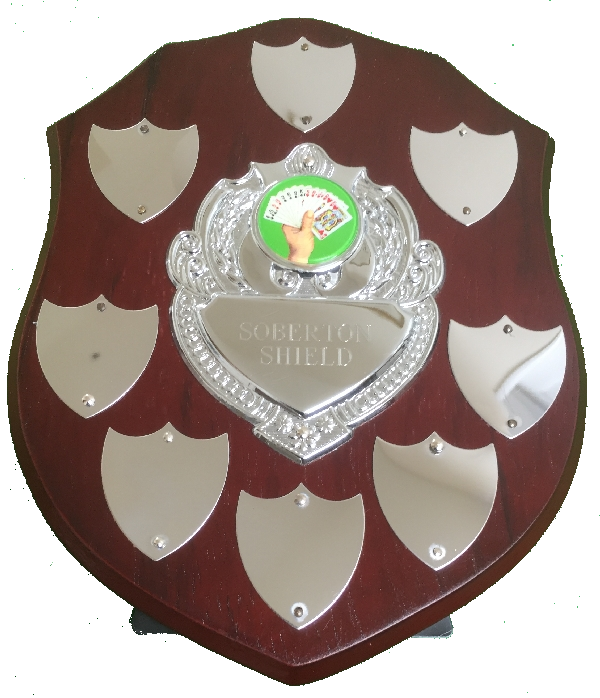# Soberton Shield Results - 2019

Team Jan 4 Mar 1 Apr 5 Oct 4 Nov 1 Dec 6 Total
1 Lord, Ladies And Gentlemen (1) -25 -12 = -37 20 -8 = 12 +34 -20 = 14 +14 +0 = 14 19 -12 = 7 42 -16 = 26 36
2 Five Queens And A Knave (2) -8 +20 = 12 17 +16 = 33 -51 +24 = -27 -42 +20 = -22 -9 +20 = 11 23 +28 = 51 58
3 Six Hearts (3) -14 -12 = -26 -15 -8 = -23 +28 -20 = 8 +56 -16 = 40 -13 +0 = -13 0 +0 = 0 -14
4 Acolytes (4) 85 -8 = 77 -11 -8 = -19 +34 -12 = 22 +33 -4 = 29 -4 -8 = -12 25 -8 = 17 114
5 Ruffs (5) -22 +32 = 10 -7 +28 = 21 0 +12 = 12 -63 +12 = -51 -10 +12 = 2 9 +24 = 33 27
6 Come Up Trumps (6) -11 -12 = -23 -2 +0 = -2 +7 -12 = -5 +6 -4 = 2 39 -20 = 19 -15 -20 = -35 -44
7 Sparkling Diamonds (7) -46 +24 = -22 -33 +12 = -21 -31 +24 = -7 -43 +28 = -15 -53 +24 = -29 -40 +28 = -12 -106
8 Six Diamonds (8) 41 -20 = 21 31 -8 = 23 -21 +0 = -21 +39 -4 = 35 31 -12 = 19 -44 +4 = -40 37
Total IMPs 126 - 126 = 0 68 - 68 = 0 103 - 103 = 0 148 - 148 = 0 89 - 89 = 0 99 - 99 = 0
No Boards

Under each date for each team is a sum which represents 'IMPs' + 'Handicap' = 'Total'.
The row 'Total IMPs' shows the 'sum of plus scores' - 'the sum of minus scores' = X where X should be zero.

Click on a table heading to sort the column under it.